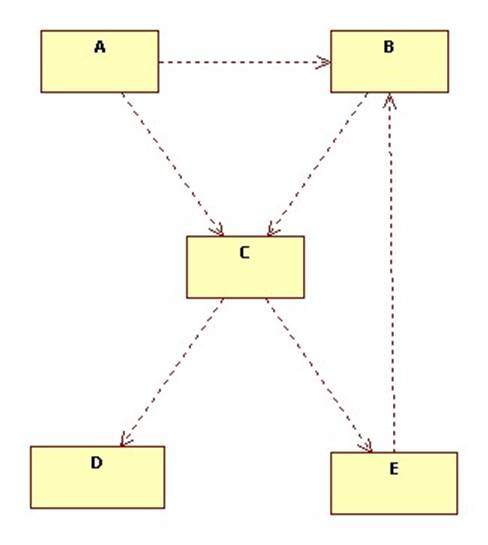# Dependency Graphs

Class A depends on a class B if changes to B could require changes to A.

Dependency is a transitive relationship:

1. A depends on A
2. If A depends on B and B depends on C, then A depends on C

Also:

3. If A references B, then A depends on B

## A references B

Class A reference class B explicitly if B appears in the declaration of A. For example, A might extend B, have a field of type B, or have a method that references B.

A method references B if the method has a parameter or local variable of type B.

Here are some examples of class A referencing class B in Java:

class A extends B {
private B field1;
private B[] field2;
public void meth1(B param1) { ... }
public void meth2() {
B local1;
...
}
}

Things can get more complicated in C++:

class A: public B {
private:
list<B> field1;
B field2[];
public:
void meth1(B* param1) { ... }
void meth2() {
B& local1;
...
}
};

It is also possible for A to implicitly reference B. This means A uses an object of type B without naming it. For example:

class B {
public void foo() { ... }
}

class C {
private B field;
public B getField() { return field; }
}

class A {
private C c;
public void meth() {
c.getField().foo();
}
}

In general, implicit references should be avoided. For example here's a slight improvement:

class A {
private C c;
public void meth() {
B b = c.getField();
b.foo();
}
}

Better still:

class A {
C c;
void meth() {
c.foo();
}
}

Then modify C to hide its field:

class C {
B field;
//B getField() { return field; }
void foo() { field.foo(); }
}

## Dependency in UML

In UML we can indicate that A references B (implicitly or explicitly) using the dependency arrow: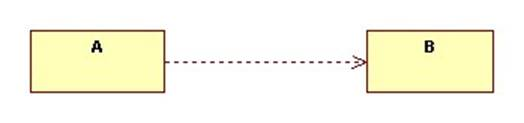Specialization and association are special cases of dependency: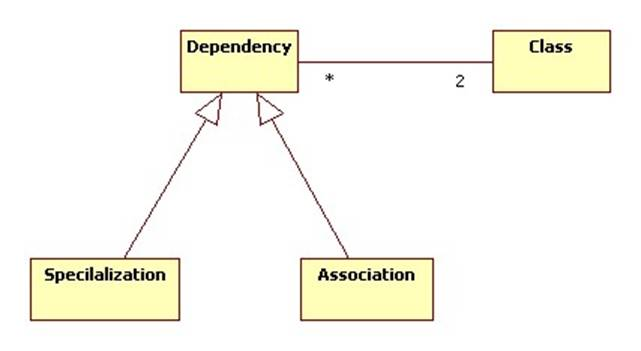Be careful, the UML dependency arrow represents our references relationship, not our dependency relationship, which is the transitive closure of the references relationship.

In other words, if A references B and B references C: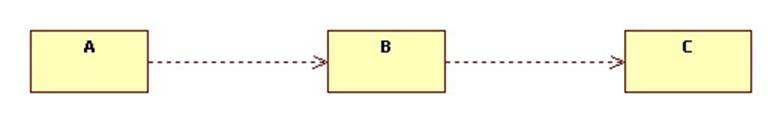Then A depends on C because changes to C may cause changes to B which may cause changes to A, but A may not reference C. Accordingly, we would not draw a dependency arrow from A to C.

## Packages

We can extend the idea of dependency and referencing to packages: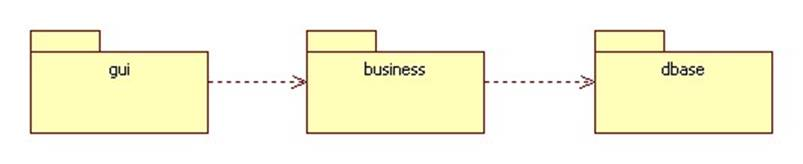In this diagram the gui package references items (classes, interfaces, sub-packages, etc.) declared in the business package, while the business package references items declared in the dbase package.

If package p1 references package p2 we say that p1 imports from p2 and that ps exports to p1.

Although the following discussion will center around classes, most of it can be applied to packages, too.

## Terminology

If class (or package) A references class (or package) B, we say that A is the client and B is the provider: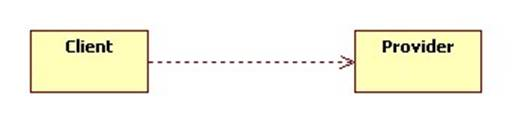Of course B may also reference A. In other words, there may be a bi-directional dependency between A and B: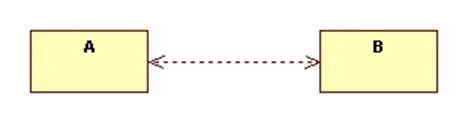In this case sometimes A is the client of B and sometimes A is the provider of B. We say that A and B are peers.

## Dependency Graphs

Let p be a program or a package. In the dependency graph of p—DG(p)—the nodes are the classes and interfaces in p. A (dashed) arrow connects node A to node B if A references B.

A class diagram for p is a special case of DG(p).

### Example

Assume package p contains the classes A, B, C, D, and E:

package p;

class A extends C {
B field1;
...
}

class B {
C field2;
...
}

class C extends D {
E field2;
...
}

class D { ... }

class E extends B {
...
}

Here's DG(p):